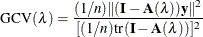# The TPSPLINE Procedure

### Penalized Least Squares Estimation

Penalized least squares estimation provides a way to balance fitting the data closely and avoiding excessive roughness or rapid variation. A penalized least squares estimate is a surface that minimizes the penalized squared error over the class of all surfaces that satisfy sufficient regularity conditions.

Define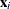as a d-dimensional covariate vector from an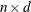matrix,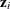as a p-dimensional covariate vector, andas the observation associated with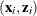. Assuming that the relation betweenandis linear but the relation betweenandis unknown, you can fit the data by using a semiparametric model as follows: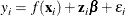where f is an unknown function that is assumed to be reasonably smooth,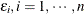, are independent, zero-mean random errors, andis a p-dimensional unknown parameter vector.

This model consists of two parts. The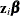is the parametric part of the model, and theare the regression variables. The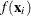is the nonparametric part of the model, and theare the smoothing variables. The ordinary least squares method estimatesandby minimizing the quantity: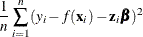However, the functional space of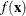is so large that you can always find a function f that interpolates the data points. In order to obtain an estimate that fits the data well and has some degree of smoothness, you can use the penalized least squares method.

The penalized least squares function is defined as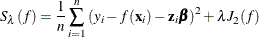where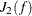is the penalty on the roughness of f and is defined, in most cases, as the integral of the square of the second derivative of f.

The first term measures the goodness of fit and the second term measures the smoothness associated with f. Theterm is the smoothing parameter, which governs the tradeoff between smoothness and goodness of fit. Whenis large, it more heavily penalizes rougher fits. Conversely, a small value ofputs more emphasis on the goodness of fit.

The estimate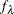is selected from a reproducing kernel Hilbert space, and it can be represented as a linear combination of a sequence of basis functions. Hence, the final estimates of f can be written as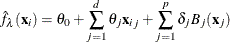where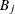is the basis function, which depends on where the data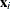are located, and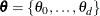and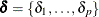are the coefficients that need to be estimated.

For a fixed, the coefficients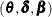can be estimated by solving ansystem.

The smoothing parameter can be chosen by minimizing the generalized cross validation (GCV) function.

If you write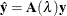then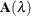is referred to as the hat or smoothing matrix, and the GCV function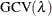is defined as